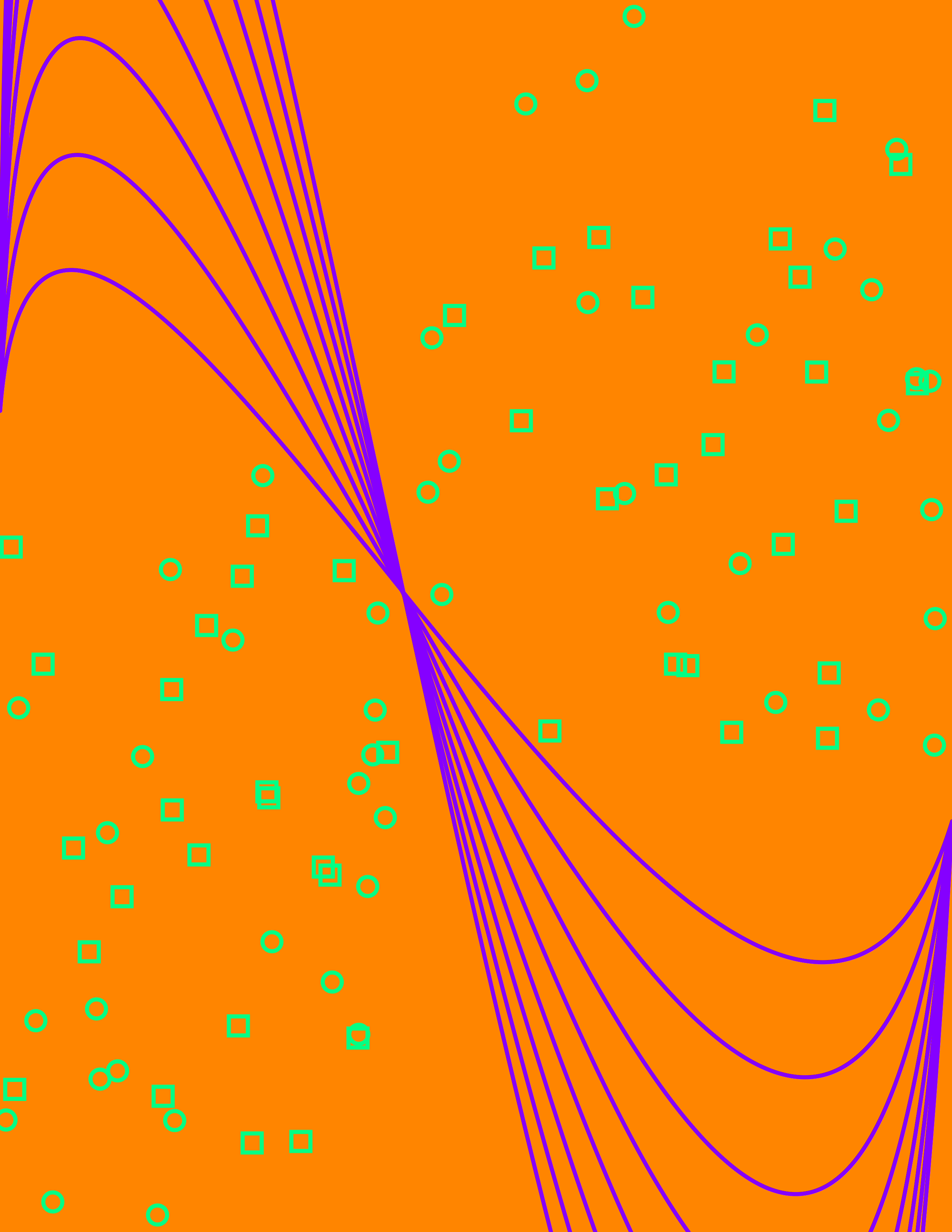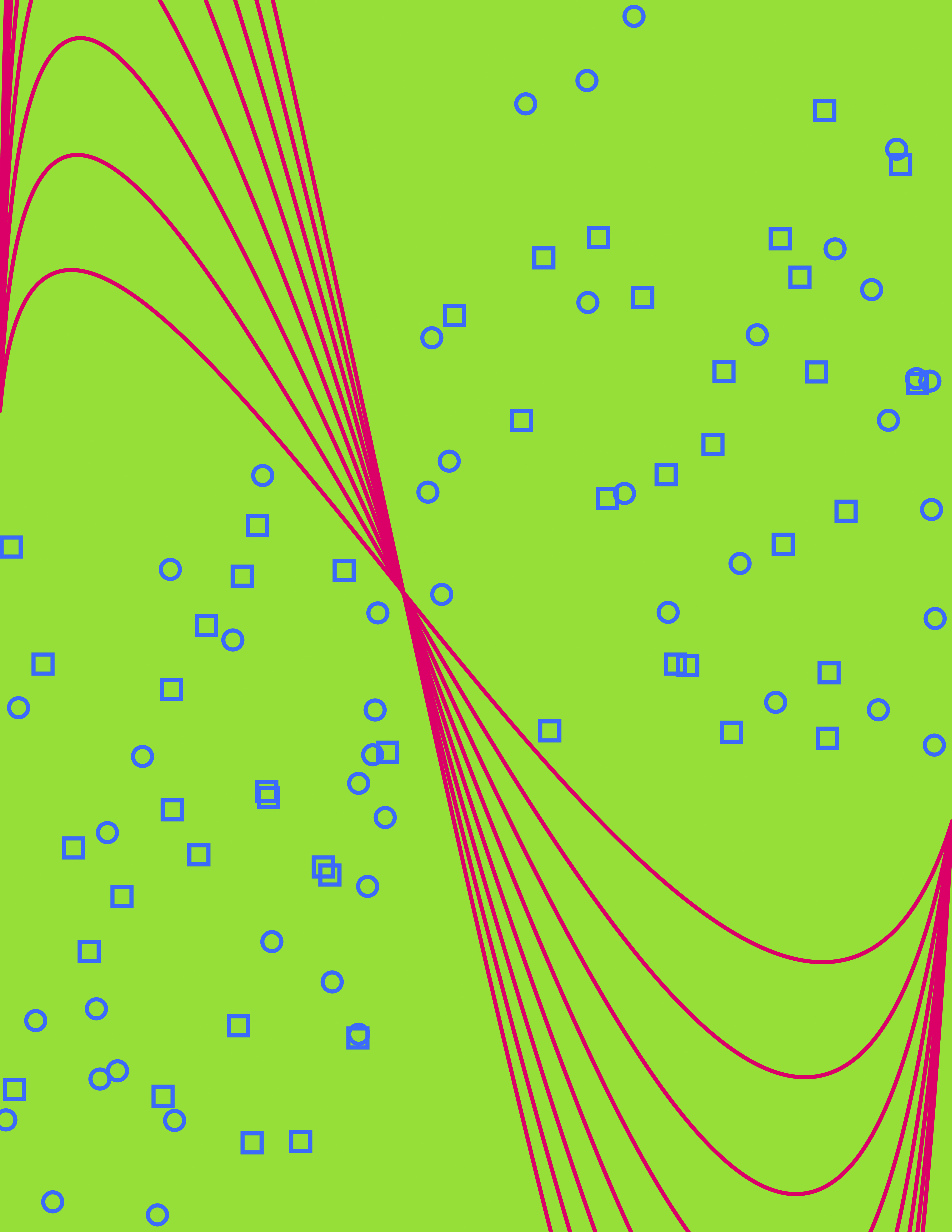# My Identity as a Poster

In Printing Code, we discussed computational color schemes, which allow designers to select a set of colors that work together without clashing. The follow-up assignment was to design a generative poster that serves as an abstract representation of my identity and uses one of the color schemes discussed in class.

Math, especially calculus, has played a significant role in my studies, so I started my design with the shape of an integral. I played with Bezier curves in Photoshop until I figured out how to approximate an integral sign with points in Processing. I then flipped it and turned it sideways, because I thought it looked better compositionally. Then I put it into a for loop so that I could draw many of them.

The spangles that cross the other diagonal of the image represent my sense of wonderment at the world and the bursts of creative energy that define my artistic side. I tried several different values of randomSeed() until I found one that spread the spangles in a way that I liked. There was one square that landed on the integral curves, and that annoyed me, so I tweaked the value of the boundaries until I could push it out of that area.

I chose a triadic color scheme, and designed my program to generate the design with a new set of triadic colors every time it runs. My favorite design (and the one that I printed ultimately) was this one:Here are a couple of alternate versions that I also liked but did not print:This is the code:

```1 2 3 4 5 6 7 8 9 10 11 12 13 14 15 16 17 18 19 20 21 22 23 24 25 26 27 28 29 30 31 32 33 34 35 36 37 38 39 40 41 42 43 44 45 46 47 48 49 50 51 52 53 54 55 56 57 58 59 60 61 62 63 64 65 66 67 68 //Kim Ash //Printing Code //Identity/Color - abstract representation of my identity using randomized computational color   PGraphics canvas; int canvas_width = 5100; int canvas_height = 6600;   float ratioWidth = 1; float ratioHeight = 1; float ratio = 1;   void setup() { size(510, 660);   canvas = createGraphics(canvas_width, canvas_height);   calculateResizeRatio();   canvas.beginDraw(); canvas.colorMode(HSB, 360, 100, 100); float h = random(100); canvas.background(h, random(100), random(100)); canvas.smooth(); canvas.noFill();   //integral shapes canvas.stroke(((h+240) % 360), 100, 100); canvas.strokeWeight(height/30); canvas.beginShape(); for(int i=2; i<10; i++) { canvas.bezier(0, canvas.height/3, (canvas.width/10)/i, 4*canvas.height/8-canvas.height/3*i, 8*canvas.width/10, 4*canvas.height/8 + canvas.height/3*i, canvas.width, 2*canvas.height/3); } canvas.endShape();   //spangles randomSeed(31); canvas.stroke(((h+120) % 360), 100, 100); for(int i=0; i<23; i++) { canvas.rect(random(canvas.width/2-canvas.width/15, canvas.width), random(2*canvas.height/3-4*canvas.width/50), canvas.width/50, canvas.width/50); canvas.ellipse(random(canvas.width/2-canvas.width/15, canvas.width), random(2*canvas.height/3), canvas.width/50, canvas.width/50); }   for(int i=0; i<23; i++) { canvas.rect(random(canvas.width/2-canvas.width/15), random(canvas.height/3, canvas.height), canvas.width/50, canvas.width/50); canvas.ellipse(random(canvas.width/2-canvas.width/15), random(canvas.height/3, canvas.height), canvas.width/50, canvas.width/50); } canvas.endDraw();   float resizedWidth = (float) canvas.width * ratio; float resizedHeight = (float) canvas.height * ratio; //displays canvas onscreen image(canvas, (width/2) - (resizedWidth/2), (height/2) - (resizedHeight/2), resizedWidth, resizedHeight);   canvas.save("colorID.tiff"); }     /* resizing function */ void calculateResizeRatio() { ratioWidth = (float) width / (float) canvas.width; ratioHeight = (float) height / (float) canvas.height;   if(ratioWidth < ratioHeight) ratio = ratioWidth; else ratio = ratioHeight; }```